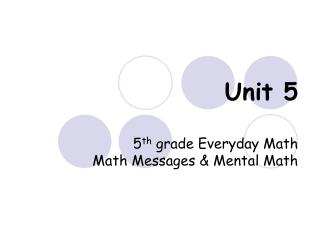DownloadDownload PresentationUnit 5 5 th grade Everyday Math Math Messages & Mental Math

# Unit 5 5 th grade Everyday Math Math Messages & Mental Math

Download Presentation## Unit 5 5 th grade Everyday Math Math Messages & Mental Math

- - - - - - - - - - - - - - - - - - - - - - - - - - - E N D - - - - - - - - - - - - - - - - - - - - - - - - - - -
##### Presentation Transcript

1. Unit 55th grade Everyday MathMath Messages & Mental Math

2. Math Message 5.1 • Write any five fractions on your slate. Circle the greatest fraction and the least fraction in your set of fractions. • An hour is what fraction of a day? • A minute is ____ of an hour? • A second is ____ of a minute? • Four days is ____ of a week? • ½ hour is how many minutes? • ¾ of an hour is ____ minutes • 1/3 of an hour is ____ minutes? • 1/6 of an hour is ____ minutes?

3. Math Message 5.2 • Take the following pattern blocks: 2 yellow hexagons, 2 red trapazoids, 3 blue rhombuses, and 6 green triangles. • If a trapezoid is worth ½, what is a rhombus worth? • What fraction of an hour is • 30 minutes? • 10 minutes? • 45 minutes? • 15 minutes? • 50 minutes? • 40 minutes? • How many hours is: • 120 minutes? • 150 minutes? • 90 minutes? • 75 minutes?

4. Math Message 5.3 • Complete problems 1-5 on journal page 131. • Look at a ruler (or geometry template) • Find 2 ½ inches on the ruler. How many ½ inches is that? • Find 3 ¼ inches on the ruler. How many ¼ inches is that? • Find 1 3/8 on your ruler. How many 1/8 inches is that? • Find 2 4/8 on your ruler. How many quarter inches is that?

5. Math Message 5.4 • Lisa has a 50-cent piece. Jamal has two quarters. Sam has 5 dimes. Hunter has ten nickels. Elliot has 50 pennies. Write a fraction to show what part of a dollar each person has. Who has the most money? • Write the following fractions as decimals: • 6/10 • 3/5 • 2/3 • 4/8 • 9/12 • 9/25

6. Math Message 5.5 • Write three decimals between each of the following pairs: • 45 seconds and 46 seconds • 7 dimes and 8 dimes • 9.32 inches and 9.33 inches • Write each number as a fraction or mixed number and then as a decimal: • Seven-tenths • Six hundredths • Three and nine-tenths • Three point nine • Six and nine-hundredths • Seven hundred eight thousandths • Forty-five hundredths • Nineteen-thousandths • Six and nineteen-thousandths

7. Math Message 5.6 • How would you use the Probability Meter to show someone what 1/8 dollar is worth? • Answer each of the following questions – use the Probability Meter if you need to • Which is greater, 6/10 or 5/8? • Which is greater 3/8 or 1/3? • Is 7/8 closer to 7/10 or to 1? • Is 1/6 closer to ¼ or to 0? • Is 0.36 closer to ¼ or to ½? • Is 0.73 closer to 0.6 or 0.9? • What fraction is about equal to 0.88? • What fraction is about equal to 0.57? • What fraction is about equal to 0.06?

8. Math Message 5.7 • Write 7/16 as a decimal. • There are 12 counters in a set. How many counters in ¾ of a set? • There are 18 counters in a set. How many counters are there in 2/9 of the set? • There are 24 counters in a set. How many counters are there in 5/6 of the set? • There are 12 counters in 2/3 of a set. How many counters are there in the whole set? • There are 15 counters in 3/5 of a set. How many counters are there in the whole set?

9. Math Message 5.8 • Using your calculator, find a way to rename 4/7 as a percent. Do not use your percent key.

10. Math Message 5.9 • Answer Problem 1 on journal page 152. • Write decimal and percent equivalents for these fractions without using a calculator: • ¼ • ¾ • 3/5 • 14/100 • 6/100 • 7/20

11. Math Message 5.10 • Look at the circle graph in problem 1 on journal page 155. For each piece of the graph, estimate what fraction, and what percent of the whole circle, it is.

12. Math Message 5.11 • Turn to problem 2 on journal page 152. Copy the number of votes for each snack in to the second column of the table on journal page 160. Leave the rest of the table blank for now. • Rename these percents as fractions. Try to find denominators that are less than 100. • 50% • 40% • 33 1/3% • 25% • 20% • 80%

13. Math Message 5.12 • Read pages 318-320, “School,” in the American Tour section of you SRB. • Write equivalent decimal and percents for these fractions: • ½ • 2/5 • 7/20 • ¾ • ¼ • 9/25 • 35/50 • 1/8 • 2/3

14. Math Message 5.13 • Complete the Time to Reflect questions on page 167.